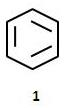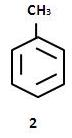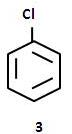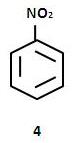Courses

# AIIMS Chemistry Mock Test - 9

## 60 Questions MCQ Test AIIMS Mock Tests & Previous Year Papers | AIIMS Chemistry Mock Test - 9

Description
This mock test of AIIMS Chemistry Mock Test - 9 for NEET helps you for every NEET entrance exam. This contains 60 Multiple Choice Questions for NEET AIIMS Chemistry Mock Test - 9 (mcq) to study with solutions a complete question bank. The solved questions answers in this AIIMS Chemistry Mock Test - 9 quiz give you a good mix of easy questions and tough questions. NEET students definitely take this AIIMS Chemistry Mock Test - 9 exercise for a better result in the exam. You can find other AIIMS Chemistry Mock Test - 9 extra questions, long questions & short questions for NEET on EduRev as well by searching above.
QUESTION: 1

Solution:
QUESTION: 2

Solution:
QUESTION: 3

### Irrespective of the source, pure sample of water always yields 88.89% mass of oxygen and 11.11% mass of hydrogen. This is explained by the law of

Solution:
QUESTION: 4

Six carbon atoms of benzene are of

Solution:
QUESTION: 5

Bromobenzene reacts with Mg in dry ether to give a compound A, which further reacts with ethanol to yield

Solution:
QUESTION: 6

The catalyst used in Friedel Craft's reaction is

Solution:
QUESTION: 7

For a first-order reaction, half life period is independent of

Solution:
QUESTION: 8

The internal energy of a subtance does not depend upon

Solution:
QUESTION: 9

The compound used as refrigerant is

Solution:
QUESTION: 10

Which of the following gas changes lime water in milky colour?

Solution:
QUESTION: 11

The shape of IF7 molecule is

Solution:
QUESTION: 12

The speeds of reactions between ions in solution__

Solution:
QUESTION: 13

In the following question, a Statement of Assertion (A) is given followed by a corresponding Reason (R) just below it. Read the Statements carefully and mark the correct answer-
Assertion(A): Industrial method of preparation of H₂O₂ involves 2-ethylanthra quinol.
Reason(R): Process becomes cyclic.

Solution:
QUESTION: 14

In the following question, a Statement of Assertion (A) is given followed by a corresponding Reason (R) just below it. Read the Statements carefully and mark the correct answer-
Assertion(A): Aqueous solution of FeCl3 is acidic in nature.
Reason(R): The hydrated form of FeCl3 consists of six water molecules FeCl3.6H2O.

Solution:
QUESTION: 15

In the following question, a Statement of Assertion (A) is given followed by a corresponding Reason (R) just below it. Read the Statements carefully and mark the correct answer-
Assertion(A): s-orbital cannot accomodate more than two electrons.
Reason(R): s-orbital does not contain any node.

Solution:
QUESTION: 16

In the following question, a Statement of Assertion (A) is given followed by a corresponding Reason (R) just below it. Read the Statements carefully and mark the correct answer-
Assertion(A): Second ionisation energy of C,N,O,F, increases in the order. C < N < F < O.
Reason (R): Ionisation energy increase with deceasing size.

Solution:
QUESTION: 17

In the following question, a Statement of Assertion (A) is given followed by a corresponding Reason (R) just below it. Read the Statements carefully and mark the correct answer-
Assertion(A): Thermoplastics can be reheated and reshaped if desired.
Reason(R): Thermoplastics have crossed links.

Solution:
QUESTION: 18

In the following question, a Statement of Assertion (A) is given followed by a corresponding Reason (R) just below it. Read the Statements carefully and mark the correct answer-
Assertion(A):An electron jumps from 5th to 2nd shell,number of possible transition state = 3.
Reason(R): Possible transition state
= ∑Δn = ∑5 − 2 .

Solution:
QUESTION: 19

In the following question, a Statement of Assertion (A) is given followed by a corresponding Reason (R) just below it. Read the Statements carefully and mark the correct answer-
Assertion(A): Water is an amphoteric solvent.
Reason(R): Water has a tendency to accept and donate a proton easily.

Solution:
QUESTION: 20

In the following question, a Statement of Assertion (A) is given followed by a corresponding Reason (R) just below it. Read the Statements carefully and mark the correct answer-
Assertion(A): [Fe(CN)6]3- is weakly paramagnetic while [Fe(CN)6]4- is diamagnetic.
Reason(R): [Fe(CN)6]3- has +3 oxidation state while [Fe(CN)6]4- has +2 oxidation state.

Solution:
QUESTION: 21

In the following question, a Statement of Assertion (A) is given followed by a corresponding Reason (R) just below it. Read the Statements carefully and mark the correct answer-
Assertion(A): Esters which contain α-hydrogens undergo Claisen condensation.
Reason(R): LiAlH4 reduction of esters gives acids.

Solution:
QUESTION: 22

In the following question, a Statement of Assertion (A) is given followed by a corresponding Reason (R) just below it. Read the Statements carefully and mark the correct answer-
Assertion(A): Carbondioxide has greater value of root mean square velocity V(rms) than Carbon monoxide.
Reason(R): V(rms)  is directly proportional to molar mass.

Solution:
QUESTION: 23

In the following question, a Statement of Assertion (A) is given followed by a corresponding Reason (R) just below it. Read the Statements carefully and mark the correct answer-
Assertion(A): Oils contain higher proportion of glycerides of saturated fatty acids.
Reason(R): Oils contain a higher percentage of olein.

Solution:
QUESTION: 24

In the following question, a Statement of Assertion (A) is given followed by a corresponding Reason (R) just below it. Read the Statements carefully and mark the correct answer-
Assertion(A): If two moles of AgNO3 and 2 moles Cu are reacted , then AgNO3 is the limiting reactant.
Reason(R): AgNO3 is left in excess and copper is consumed fully.

Solution:
QUESTION: 25

In the following question, a Statement of Assertion (A) is given followed by a corresponding Reason (R) just below it. Read the Statements carefully and mark the correct answer-
Assertion(A): Superoxides of alkali metals are paramagnetic.
Reason(R): Superoxides contain the ion O⁻2 which has one unpaired electron.

Solution:
QUESTION: 26

In the following question, a Statement of Assertion (A) is given followed by a corresponding Reason (R) just below it. Read the Statements carefully and mark the correct answer-
Assertion(A): Tetracycline is a will known broad spectrum antibiotic.
Reason(R): It can kill large number of bacteria of gram positive as well as gram negative stains.

Solution:
QUESTION: 27

In the following question, a Statement of Assertion (A) is given followed by a corresponding Reason (R) just below it. Read the Statements carefully and mark the correct answer-
Assertion(A): Hydrogen gas cannot be prepared in laboratory.
Reason(R): Hydrogen gas of high purity is obtained by electrolysing warm aqueous barium hydroxide between nickel electrodes.

Solution:
QUESTION: 28

In the following question, a Statement of Assertion (A) is given followed by a corresponding Reason (R) just below it. Read the Statements carefully and mark the correct answer-
Assertion(A): Sodium reacts with oxygen to form Na₂O₂ whereas potassium reacts with oxygen to form KO₂.
Reason(R): Potassium is more electro-positive than sodium.

Solution:
QUESTION: 29

In the following question, a Statement of Assertion (A) is given followed by a corresponding Reason (R) just below it. Read the Statements carefully and mark the correct answer-
Assertion(A): According to Graham's law, rate of diffusion of gas A is directly related to square root of its molar mass.
Reason(R): Rate of effusion of hydrogen is four times to that of oxygen.

Solution:
QUESTION: 30

In the following question, a Statement of Assertion (A) is given followed by a corresponding Reason (R) just below it. Read the Statements carefully and mark the correct answer-
Assertion(A): Mesomeric and inductive effects are permanent.
Reason(R): Electromeric effect is temporary.

Solution:
QUESTION: 31

In the following question, a Statement of Assertion (A) is given followed by a corresponding Reason (R) just below it. Read the Statements carefully and mark the correct answer-
Assertion(A): According to the principle of common ion effect , the solubility of HgI2 is expected to be less in an aqueous solution of KI than in water. but HgI2 dissolves in an aqueous solution of KI to form a clear solution.
Reason(R): Iodine ion, I-, is highly polarisable.

Solution:
QUESTION: 32

A current liberates 0.504 g of hydrogen in 2 hours, the amount of copper liberated from a solution of CuSO₄ by the same current flowing for the same time would be

Solution:
QUESTION: 33

In the following question, a Statement of Assertion (A) is given followed by a corresponding Reason (R) just below it. Read the Statements carefully and mark the correct answer-
Assertion(A): Electron withdrawing groups in aryl halides decrease the reactivity towards nucleophilic substitution.
Reason(R): 2,4-dinitrochlorobenzene is less reactive than chlorobenzene.

Solution:
QUESTION: 34

If the root mean square (r.m.s.) velocity of a gas is doubled, then its temperature is

Solution:
QUESTION: 35

Which of the following is the use of electrolysis?

Solution:
QUESTION: 36

Which of the following has largest size?

Solution:
QUESTION: 37

Fires, that results from the combustion of alkali metals can be extinguished by

Solution:
QUESTION: 38

In electrolysis of NaCl when Pt electrode is taken then H2 is liberated at cathode while with Hg cathode it forms sodium amalgam. The reason for this is

Solution:

In electrolysis of NaCl when Pt electrode is taken then H2 liberated at cathode while with Hg cathode it forms sodium amalgam because more voltage is required to reduce H+ at Hg than Pt

QUESTION: 39

which of the following is formed, when barium reacts with oxygen?

Solution:
QUESTION: 40

A 500g toothpaste sample has 0.2g fluoride concentration. What is the concentration of F- ions in terms of ppm level?

Solution:
QUESTION: 41

Heavy water is used in nuclear reactors as

Solution:
QUESTION: 42

Carboxylic acids readily dissolve in aqueous sodium bicarbonate , liberating carbon dioxide . When one of the following is correct ?

Solution:
QUESTION: 43

Which of the following is a strong electrolyte?

Solution:
QUESTION: 44

The best explanation for the solubility of MnS in dil. HCl is that

Solution:
QUESTION: 45

The compound whose 0.1 M solutions is basic is

Solution:
QUESTION: 46

Ketones (R₁COR₂);R₁═R₂═alkyl group, can be obtained in one step by

Solution:
QUESTION: 47

Which does not contain conjugated system?

Solution:

In dienes, when the double bonds connected to each other by a single bond, this arrangement is known as conjugated.

QUESTION: 48

Identify the correct order of reactivity in electrophilic substitution action of the following compounds.Solution:
QUESTION: 49

Which of the following has the most acidic hydrogen ?

Solution:
QUESTION: 50

Which of the following is the example of SN2 reaction?

Solution:
QUESTION: 51

Select incorrect statement :

Solution:
QUESTION: 52

Which of the following is the second most abundant element in the earth's crust?

Solution:
QUESTION: 53

The co-ordination number of a metal crystallising in a hexagonal close packing structure is

Solution:
QUESTION: 54

Quartz is a crystalline variety of

Solution:
QUESTION: 55

What is the normality of a 1 M solution of H₃PO₄ ?

Solution:
QUESTION: 56

Which of the following should be done in order to prepare 0.40 M NaCl starting with 100 ml. of 0.30 M NaCl (mol.wt.of NaCl=58.5)

Solution:
QUESTION: 57

How many litres of CO2 at STP will be formed when 100ml. of 0.1 M H2SO4 reacts with excess of Na2CO3?

Solution:
QUESTION: 58

X litres of CO is present at STP. It is completely oxidised to CO2. The volume ofCO2 formed is 11.2 litres at STP. What is the value of X.

Solution:
QUESTION: 59

Arrange in order of decreasing trend towards ES (electrophilic substitution) reactions: Chlorobenzene (I), Benzene (II), Anilinium chloride (III), Toluene (IV)

Solution:
QUESTION: 60

In acidic medium, KMnO₄ oxidised FeSO₄ solution. Which of the following statements is correct ?

Solution: